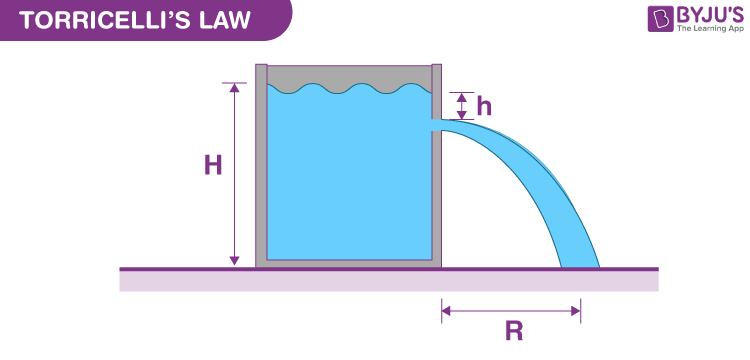# Torricelli's lawTorricelli’s law has practical applications in daily life. The physical law describes a major relationship between liquid exit velocity and its height in the container. You will know about this relationship and how to calculate exit velocity by applying Torricelli’s theorem in this article.

### Torricelli’s Theorem

Torricelli had interests in various aspects of physics and mathematics and torricelli’s theorem was one of his biggest achievements. The law explains the relation between fluid leaving a hole and the liquid’s height in that container.

The relationship can be summarized in the following manner. If you have a container filled with fluid with small holes at the bottom of the container, the fluid leaves through the hole with velocity same as it would experience if dropped from the same height to the hole level. If the liquid is dropped from a height “h”, it would have a velocity v and this “v” is the same velocity at which the liquid leaves the hole when the height of fluid “h” is same as the liquid dropped in the container.

We also assume that it is an ideal fluid which means that the liquid is incompressible, non-viscous and laminar flow. These factors cannot be neglected since the same rules cannot be applied to non-fluids as their viscosity and flow may not be the same across the liquid itself.

### Torricelli’s Law Derivation

Assuming that the fluid is incompressible, Bernoulli’s principle states that:

/2 + gh + P/ρ = constant

Where,

v is speed of liquid,

g denotes gravitational acceleration,

h shows liquid’s height over reference point,

ρ is density.

P is equal to atmospheric pressure at the top of the container.

“v” is considered as “0” because the liquid surface drops in height slowly compared to the speed at which liquid leaves the tank.

“h” is 0 and “p” is atmospheric pressure at opening h = 0.

$$\begin{array}{l}gh+\frac{p_{atm}}{ρ} = \frac{v^{2}}{2} + \frac{p_{atm}}{ρ}\end{array}$$

v² = 2gh

V = √(2gh)

A simple experiment to test Torricelli’s law can also be performed by a soda bottle by puncturing the bottom with a small hole. As the height in the reservoir decreases, the exit velocity decreases.

## Frequently Asked Questions – FAQs

Q1

### What is Torricelli’s formula?

Torricelli’s theorem also comes in equation form: v = √(2gh), where v is the velocity of the fluid, g is the acceleration due to gravity, and h is the height of the fluid above the hole.
Q2

### Why is Torricelli’s law important?

Torricelli had interests in various aspects of physics and mathematics, and Torricelli’s theorem was one of his most significant achievements. The law explains the relation between fluid leaving a hole and the liquid’s height in that container.
Q3

### What is an example of Torricelli’s Law?

The best illustration of Torricelli’s law is the so-called “spouting can”, where a liquid container has holes made at different heights. The depth of liquid above each hole determines the exit velocity from that hole.
Q4

### What is meant by Torricelli’s Law?

Torricelli’s law is a principle of fluid dynamics. The law describes the relationship between the exit velocity of an ideal fluid from a small hole and the depth of fluid above that hole.
Q5

### When can you use Torricelli’s equation?

Torricelli’s law can only be applied when viscous effects can be neglected, which is the case for water flowing out through orifices in vessels.

To know more about Torricelli’s theorem examples and Torricelli’s law differential equation, you can visit us at BYJU’S.

## Watch the video and understand the equation of continuity.Test Your Knowledge On Torricellis Law!